# HC Verma Solutions Class 12 Chapter 8 Gauss’s Law

HC Verma Solutions Class 12 Chapter 8 Gauss’s Law will help students find the right answers to all the exercises and important questions given in the chapter. By going through the solutions students will understand how to solve problems related to Gauss’s Law which was first formulated by Joseph-Louis Lagrange in the year 1773 and later was improvised by Carl Friedrich Gauss in the year 1813. The formula is expressed as ϕ = Q/ϵ0 where Q = total charge within the given surface and ϵ0 = the electric constant. Gauss’s law is also called as Gauss’s flux theorem. Coulomb’s law can also be derived from this theorem.

## Key Chapter Topics Related To Gauss’s Law

Some of the important topics included are;

• Solid Angle
• Application of Gauss’s Law
• Spherical Charge Distributions
• The Flux of Electric Field Through a Surface

Class 12 students and even JEE aspirants can use the HC Verma Solutions to understand the concepts more clearly as well as enhance their problem-solving skills. With the solutions, students can significantly boost their preparation and perform well in the exams. HC Verma book for physics is often regarded as the perfect book for students who are willing to prepare for Engineering and medical entrance exams. The solutions which are also available in a PDF format will give students a clear advantage over others when they have to tackle difficult numerical problems in less time.

## Class 12 Important Question In Chapter 8

1. A point charge c is placed in a metal block having a cavity. If the charge C is kept or brought outside the metal, will charge c experience any electric force?

2. A nonconducting material is used to make a circular ring of radius r and is placed with its axis parallel to a uniform electric field. The ring is then rotated about a diameter through 180°. What will happen to the flux of the electric field? Will it increase or decrease?

3. If the flux of the electric field through a spherical surface of radius 10 cm surrounding the total charge is 25 V-m then what will the flux over a concentric sphere of radius 20 cm be

(a) 200 V-m (b) 100 V-m (c) 500 V-m (d) 25 V-m

4. What happens when an electric dipole is kept at the centre of a sphere.

(a) The electric field is not zero anywhere on the sphere.

(b) The electric field is zero on a circle on the sphere.

(c) The flux of the electric field through the sphere is zero.

(d) The electric field is zero at every point of the sphere.

5. There is no net charge in a metallic particle which is placed beside a finite metal plate having a positive charge. State where the electric force will be present on the particle?

(a) parallel to the plate (b) away from the plate (c) close to the plate (d) zero electric force.

## HC Verma Solutions Vol 2 Gauss’s Law Chapter 8

Remember:

By Gauss’s law, flux of net electric field through a closed surface S equals the net charge enclosed (qin) by the surface divided by ϵ0.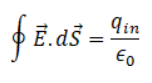Question 1: The electric field in a region is given byFind the flux of this field through a rectangular surface of area 0.2 m2 parallel to the y–z plane.

Solution:

The plane is parallel to y-z plane. The normal to this plane is parallel to x-axis. Therefore, area vector for this plane is given by 0.2m2 i

So, surface area of plane= 0.2 m2 i

Flux of electric field through a surface of area = φ = E . dS

=> φ = [(3/5)E0 i + (4/5)E0 J ] . (0.2 i) = (3/5) x 0.2 x E0

=> φ = (3/5) x 0.2 x 2.0 × 103 = 240 Nm^2/C

Question 2: A charge Q is uniformly distributed over a rod of length l. Consider a hypothetical cube of edge l with the centre of the cube at one end of the rod. Find the minimum possible flux of the electric field through the entire surface of the cube.

Solution:

Linear charge density of rod = Q/l

Where Q is total charge on the rod

Length of rode = edge of cube = l

Portion of rod inside cube = l/2

Using Gauss’s theorem, flux through the hypothetical cube is φ = (Qino)

Where Qin is the charge enclosed inside the cube

Now, charge enclosed = Qin = Q/l x l/2 = Q/2

So, φ = (Q/2/Єo) = Q/2 φ = (Qino)

Question 3: Show that there can be no net charge in a region in which the electric field is uniform at all points.

Solution:

The electric field is uniform (Given)

If we consider a plane perpendicular to electric field, we find this plane is an equipotential surface. If a test charge is introduced on the surface, then the work done will be zero in moving the test charge on this surface.

But if there is net charge is present in this region, the test charge will experience a force due to which non-zero work needs to be done to move our test charge from one point to another. This means, potential difference between these two points is finite. So this fact is contradiction to the fact that the surface is equipotential.

Therefore, net charge in the region of uniform electric field is zero.

Remember:

*Equipotential surfaces are perpendicular to the electric field lines

Question 4: The electric filed in a region is given by E = [Eox/l ] i. Find the charge contained inside a cubical volume bounded by the surfaces x = 0, x = a, y = 0, y = a, z = 0 and z = a. Take E0 = 5 × 103 NC–1, l = 2 cm and α = 1 cm.

Solution:

E = [Eox/l ] I (Given)

Edge of cube = a = 1 cm ; length = l = 2 cm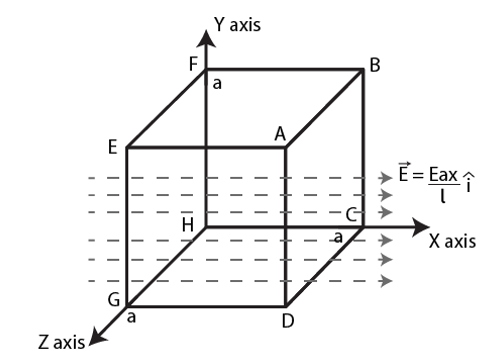From figure,

we can see that electric field lines are parallel to the normal of the surfaces ABCD and EFGH therefore flux is nonzero through these surfaces, whereas for other faces of the cube electric field is perpendicular to normal of faces therefore flux through these surfaces is zero.

At EFGH, x = 0, electric field at EFGH = 0

At ABCD, x = a, electric field at ABCD is E = Eoa/l i

The net flux through the whole cube is only through side ABCD is

φ = E . A = Eoa2/l = [5×103 x (1×10-2)2]/[2×10-2] = 25 NM2/C

Now, net charge, q = Єo φ = 8.85 x 10-12 x 25 = 2.2125 x 10-12 C

Question 5: A charge Q is placed at the centre of a cube. Find the flux of the electric field through the six surfaces of the cube.

Solution:

Total charge in the cube = Q at the center.

From Gauss’s law we can conclude that total flux enclosed by the cube : φ = Qino

= Q/Єo

Since charge is placed at centre it is placed symmetrically with respect to all the faces of the cube. So, equal flux passes through all the six surfaces.

Flux passing through each surface = φ0 = φ/6

=> φ0 = Q/6Єo

Question 6: A charge Q is placed at a distance a/2 above the centre of a horizontal, square of edge a as shown in figure. Find the flux of the electric field through the square surface.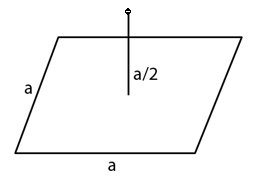Solution:

Length of square edge = a

Area of the plane = a2

Distance of charge Q above the center = a/2

Since charge is placed at centre it is placed symmetrically with respect to all cube faces. So, equal flux passes through all the six surfaces.

Flux passing through each surface = φ0 = Q/6Єo

The flux through the given surface is Q/6Єo

Question 7: Find the flux of the electric field through a spherical surface of radius R due to a charge of 10–7 C at the centre and another equal charge at a point 2R way from the centre.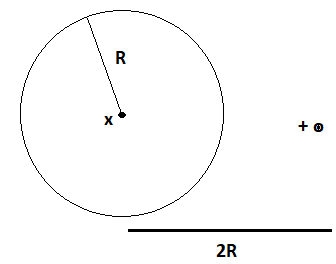Solution:

Magnitude of charges = 10-7C

By Gauss’s law, flux of net electric field through a closed surface equals the net charge enclosed by the surface divided by ϵ0

The only charge located inside the sphere will contribute to the flux passing through the sphere.

φ = ∮ E . ds = Q/ ϵ0 = 10-7/[8.85×10-12] = 1.1 x 104 Nm2C-1

Question 8: A charge Q is placed at the centre of an imaginary hemispherical surface. Using symmetry arguments and the Gauss’s law, find the flux of the electric field due to this charge through the surface of the hemisphere (figure).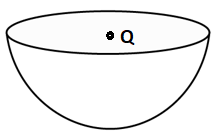Solution:

Consider charge Q is at the centre.

Net charge enclosed by the sphere Qin = Q

From Gauss’s law flux of electric field through this sphere is φ = Q/ϵ0

For a hemisphere (open top), total flux through its curves surface, φ’ = Q/2ϵ0

Question 9: A spherical volume contains a uniformly distributed charge of density 2.0 × 10–4 C m–3. Find the electric field at a point inside the volume at a distance 4.0 cm from the centre.

Solution:

Volume charge density = ρ = 2.0 × 10-4 Cm-3 (given)

Let us assume that, “r” be spherical gaussian surface inside the sphere of radius, r = 4cm

And q = ρ x 4/3 πr3

Where, “q” be the Charge enclose by this gaussian spherical surface

= 2.0 × 10-4 x 4/3 πr3

All the points on the surface are equivalent and by symmetry we can find that field at every point on this surface is equal in magnitude and radial in direction. Therefore, flux through this surface is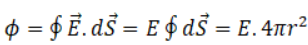By Gauss’s law, ∮ E. dS = qin0 = ρ x 4 πr3/ ϵ0

=>E . 4 πr2 = ρ x 4 πr3/ ϵ0

=>E = ρ r/ϵ0

Putting the value of ρ, r and ϵ0

E = (2×10-4) x [4×10-2]/[8.85×1012] = 3 x 105 N/C

Question 10: The radius of a gold nucleus (Z = 79) is about 7.0 × 10–15 m. Assume that the positive charge is distributed uniformly throughout the nuclear volume. Find the strength of the electric field at

(a) the surface of the nucleus and

(b) at the middle point of a radius.

Remembering that gold is a conductor, is it justified to assume that the positive charge is uniformly distributed over the entire volume of the nucleus and does not come to the outer surface?

Solution:

Charge on the gold nucleus = Q = 79 x (1.6 x 10-19) C

(a) The value of E is fixed for a particular radius: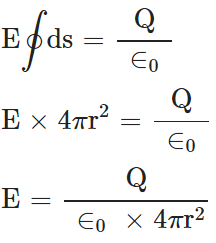=> E = [79x(1.6×10-19)]/[(8.85×10-12) x 4 x 3.14x(7×10-10)2] = 2.31513 x 1021 NC-1

(b) Radius = r = (7/2) x 10-10 m and net charge = 79 x 1.6 x 10-19 C

V = (4/3) πr3

= (4/3) x 22/7 x 343/8 x 10-30

Volume charge density = [79×1.6×10-19] /[(4/3) π x 343 x10-30]

Now, Charge enclosed by imaginary sphere of radius 3.5 x 10-10 is

= Volume charge density x V

Putting values, we get

= [79×1.6×10-19]/8

Now,

=> E = [79×1.6×10-19]/[8 x 4πЄo r2]

Here r = 3.5 x 10-10

=> E = 1.16 x 1021 NC-1

when electric charge is given to a conductor it comes on its surface. But nucleons are bound by strong nuclear force inside nucleus which holds them and prevents them from coming out of conductor. So, it is justified to assume that electric charge is uniformly distributed in its entire volume.

Question 11: A charge Q is distributed uniformly within the material of a hollow sphere of inner and outer radii r1 and r2 figure. Find the electric field at a point P a distance x away from the centre for r1 < x < r2. Draw a rough graph showing the electric field as a function of x for 0 < x < 2r2 figure.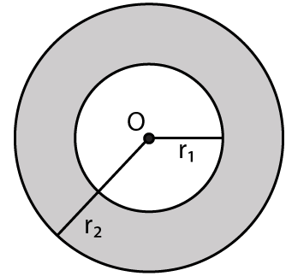Solution:

Let r1 and r2 be the Inner and outer radius of hollow sphere.

Q be the amount of charge distributed within the hollow sphere.

Volume of charge = (4/3) π (r23 – r13)

Now, density = Q/[(4/3) π (r23 – r13)]

Charge enclosed by this surface:

q = Density x volume of the part of consisting charge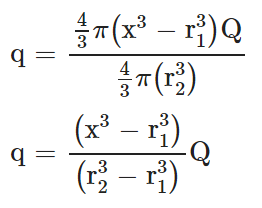By Gauss’s law:

∮E. ds = q/Єo

The surface integral is carried out on th esphere of radius x and q is the charged enclosed by this sphere.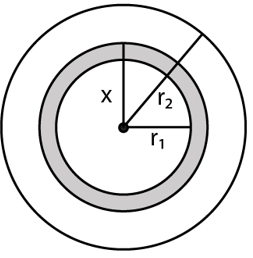E(4πx2) = q/ Єo

This electric field is directly proportional to x for r1 < x < r2

For r2 < x < 2r2

Total electric flux through this surface = (4πx2) E

or E = Q/4πx2Єo

The graph showing electric field as a function of x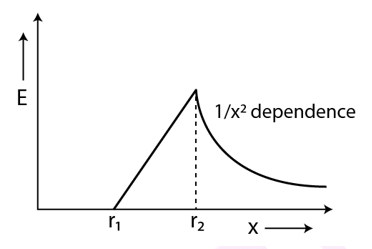Question 12: A charge Q is placed at the centre of an uncharged, hollow metallic sphere of radius a.

(a) Find the surface charge density on the inner surface and on the outer surface.

(b) If a charge q is put on the sphere, what would be the surface charge densities on the inner and the outer surfaces?

(c) Final the electric field inside the sphere at a distance x from the centre in the situations (a) and (b).

Solution:

Surface area of sphere = 4πa2

where “a” is radius of sphere.

Also, electric fields at all points inside the conductor is zero. And Q be the amount of charge present at the centre of the hollow sphere.

Due to induction, the charge induced at the inner surface be −Q and the charge induced on the outer surface is +Q

(a) σ = charge/(total surface area)

Surface charge density of the inner surface and outer surface:

σin = -Q/(4πa2) and σout = Q/(4πa2)

(b) If another charge “q” is added to the outer surface, all the charge on the metal surface will move to the outer surface. Thus, inner charge density remain same but outer charge density on the surface become Q + q.

σin = -q/(4πa2) and σout = Q+q/(4πa2)

(c) Applying Gauss law on this sphere;

∮ E. dS = Q/Єo

Where ∮ dS = 4π x2

=> E = Q/4πЄox2

Where, Q be the charge enclosed by the sphere.

For situation(b) the charge enclosed by the Gaussian surface remains the same.

[since point is inside the sphere, there is no effect of the charge q given to the shell.]

Therefore, for situations (a) and (b) the electric field inside the sphere at a distance x from the centre is same.

Question 13: Consider the following very rough model of a beryllium atom. The nucleus has four protons and four neutrons confined to a small volume of radius 10–15 m. The two 1s electrons make a spherical charge cloud at an average distance of 1.3 × 1011 m from the nucleus, whereas the two 2s electrons make another spherical cloud at an average distance of 5.2 × 10–11 m from the nucleus. Find the electric field at

(a) a point just inside the 1s cloud and (b) a point just inside the 2s cloud.

Solution:

(a) Let r1 is the gaussian spherical surface of radius just equal to the radius of 1s cloud.

Here, flux = φ = ∮E.dS = E∮ds = E. 4πr12 …(1)

Also, Charge enclosed by this sphere = total charge on the nucleus = q

q = 4 x 1.6 x 10-19 C

Now, Using gauss’s law and equation (1), we get

E. 4π r2 = q/Єo

Here, E = electric field at a point just inside the 1s cloud.

=> E = q/4πr12Єo

We are given:

Radius of 1-s charge cloud = r1 = 1.3× 10-11m

=> E = [2×1.6×10-19]/[4×3.14x(1.3× 10-11)2x8.85×10-12 = 3.4 x 1013 N/C

(b) Charge enclosed by this sphere = Total charge on the nucleus + charge of 2 1s electrons = q

q = 2 x 1.6 x 10-19 C

We have, E = q/4πr22Єo

Here, E = electric field at a point just inside the 2s cloud

E = [2×1.6×10-19]/[4×3.14x(5.2× 10-11)2x8.85×10-12 = 1.1 x 1012 N

Question 14: Find the magnitude of the electric field at a point 4 cm away from a line charge of density 2 × 10–6 C m–1.

Solution: Charge density of line charge =r = 2× 10-6 Cm-1

Distance of point from line charge = λ = 4cm = 4 × 10-2 m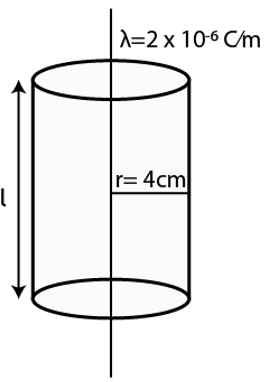Given: Radius of 2-s charge cloud = r2 = 5.2× 10-11m

The charge enclosed by the gaussian surface = λ × l

Using gauss’s law: φ = ∮E.dS = E∮ds = E. 2πrl

=> E. 2πrl = λl/ Єo

=>E = 2 x [10-6]/[2×3.14x4x10-2x8.85×10-12] NC-1 = 8.99 x 105 N/C

Which is the magnitude of electric field at a distance 4cm away from the line charge.

Question 15: A long cylindrical wire carries a positive charge of linear density 2.0 × 10–6 C m–1. An electron revolves around it in a circular path under the influence of the attractive electrostatic force. Find the kinetic energy of the electron. Note that it is independent of the radius.

Solution:

Electric field E due to a linear charge distribution of linear charge density λ at a distance r from the line

E = λ/2πЄor …(1)

And Magnitude of Force experienced by q in an electric field of intensity = F = qE

Here, charge particle is electron so the charge = q = e

As per given, qE = mv2/r

We know, K.E. = (1/2) mv2

=> (1/2) mv2 = (1/2) qE r

or K = qEr/2

Using (1), we have

K = qλ/4πЄo

Thus, kinetic energy is independent of radius.

We are given:

q = charge of electron = 1.6 × 10-19C

λ = 2.0× 10-6C and Єo = 8.85 x 10-12

On substituting values and solving, we get

K = 2.88 x 10-17 J

Question 16: A long cylindrical volume contains a uniformly distributed charge of density ρ. Find the electric field at a point P inside the cylindrical volume at a distance x from its axis.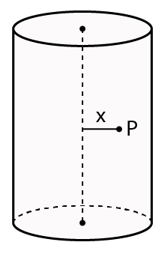Solution:

Volume charge density inside cylinder = ρ

Let h be the height of cylinder.

Therefore, charge Q at point P = ρπx2h

For electric field ∮E.ds = Q/Єo

=> E = [ρπx2h]/[2πxhЄo] = ρx/2Єo

Question 17: A nonconducting sheet of large surface area and thickness d contains uniform charge distribution of density ρ. Find the electric field at a point P inside the plate, at a distance x from the central plane. Draw a qualitative graph of E against x for 0 < x < d.

Solution: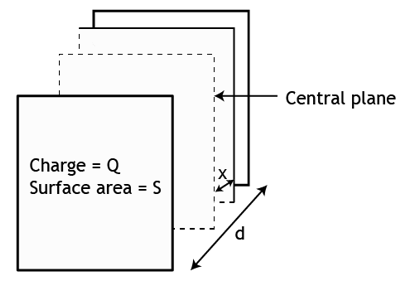Volume of this cuboidal surface = V = Ax

Total flux passing through the gaussian surface = ∮E.ds = EA

Volume charge density of the sheet = Q/sd

Where Q = charge on the sheet and s = SA of the sheet and d= sheet thickness

Now, EA = ρAx/Єo

=> E = ρx/Єo  ; valid only inside the sheet i.e. when x < d/2

For outside the sheet (when x > d/2)

EA = ρA(d/2)/Єo = ρd/2Єo

Graph of electric field for 0<x<d is as shown below: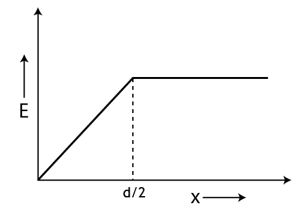Question 18: A charged particle having a charge of –2.0 × 10–6 C is placed close to a nonconducting plate having a surface charge density 4.0 × 10–6 C m–2. Find the force of attraction between the particle and the plate.

Solution:

The electric field due to a conducting thin sheet,

E = σ/2Єo

Force on a charge particle of charge q in presence of electric field is: F = qE

=> F = qσ/2Єo

The magnitude of attractive force between the particle and the plate,

F = qE

Putting values of σ and q,

F = [(-2.0 x 10-6 ) x ( 4.0 x 10-6)]/[2 x (8.55 x 10-12)]

F = 0.45 N

Therefore, force of attraction between the particle and the plate is 0.45N.

Question 19: One end of a 10 cm long silk thread is fixed to a large vertical surface of a charged nonconducting plate and the other end is fastened to a small ball having a mass of 10 g and a charge of 4.0 × 10–6 C. In equilibrium, the thread makes an angle of 60° with the vertical. Find the surface charge density on the plate.

Solution:

Mass of ball = 10g

Length of silk thread = 10cm

Charge of ball = 4.0× 10-6 C

Equilibrium angle of thread with vertical is 60°

Let σ be the surface charge density on the plate.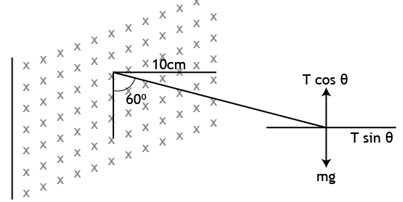There are 2 forces acting on the ball:

W = mg (weight of the ball downwards)

And

Electric force due to non-conducting plate producing electric field E = F = qE

Due to these forces, tension develops in the thread

E = σ/2 Єo

The tension force T due to string is divided into horizontal and vertical components i.e. T sin60° and T cos60°.

=> T sin60° = qE and T cos60° = mg

=> tan 60o = qE/mg

or E = mg tan60o/q

Also, elecrtic field due to plate,

E = σ/2 Єo

=> mg tan60o/q = σ/2 Єo

or σ = 2Єo mg tan60o/q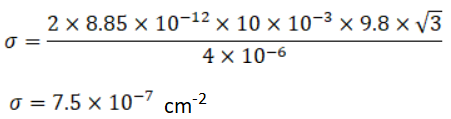Question 20: Consider the situation of the previous problem.

(a) Find the tension in the string in equilibrium.

(b) Suppose the ball is slightly pushed aside and released. Find the time period of the small oscillations.

Solution: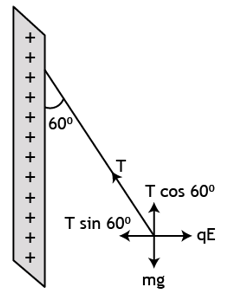There are 2 forces acting on the ball:

W = mg (weight of the ball downwards)

And

Electric force due to non-conducting plate producing electric field E = F = qE

Due to these forces, tension develops in the thread

E = σ/2Єo …(1)

The tension force T due to string is divided into horizontal and vertical components i.e. T sin60° and T cos60°.

Since the ball is in equilibrium, the net horizontal and vertical force on the ball is zero

By equilibrium along horizontal direction,

=> T sin60° = qE and T cos60° = mg

=> tan 60o = qE/mg

or E = mg tan60o/q

Also, electric field due to plate,

(1)=> σ/2Єo = mg tan60/q

=> σ = [2×8.85×10-12x10x10-3x9.8x√3]/[4×10-6]

= 7.5 x 10-7 Cm-2

(a) The tension in the string in equilibrium condition

T cos60° = mg

T = 2mg = 2 x 10 x 10-3 x 9.8 = 0.196 N

(b) When the ball will come in mean position, tension, weight and electric force will balance.

F = √[(mg)2+(qσ/2Єo) 2] [F = ma]

Time period of small oscillations = T = 2π √(l/g)

= 2π √((10×10-2/9.8)

= 0.45 s

Question 21: Two large conducting plates are placed parallel to each other with a separation of 2.00 cm between them. An electron starting from rest near one of the plates reaches the other plate in 2.00 microseconds. Find the surface charge density on the inner surfaces.

Solution:

Let “a” acceleration of electron and σ is surface charge density of the plate

Using 2nd law of motion: s = ut + (1/2) at2

Since electron starts from rest, so u = 0

=> s = (1/2) at2

=> a = 2s/t2 is the acceleration is provided by the force due to electric field between plates.

Force applied to the particle = F = ma = 2ms/t2

Also, force is provided by the electric field (E) between the plates = F = qE

=> 2ms/t2 = qE

we know, Electric field due to a conducting plate of surface charge density σ is E = σ/Єo

=> qσ/Єo = 2ms/t2

=> σ = 2msЄo/qt2 = 2 x 9.1 x 10-31 x 8.85 x 10-12/[1.6×10-19 x (2 x10-6)2] C m-2

=> σ = 0.503 x 10-12 Cm-2

Question 22: Two large conducting plates are placed parallel to each other and the carry equal and opposite charges with surface density σ as shown in figure. Find the electric field

(a) at the left of the plates.

(b) in between the plates and

(c) at the right of the plates.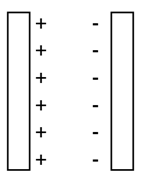Solution:

Let σ be the surface charge density on the plates.

The electric field due to plate 1 = E1 = σ/2Єo

The electric field due to plate 2 = E2 = σ/2Єo

(a) Directions of the plates will be opposite to each other on any point.

Net electric field at a point on the plates = σ/2Єo – σ/2Єo =0

(b) Direction of the fields will be same. So, total electric field:

σ/2Єo + σ/2Єo  = σ/Єo

(c) The strength of the electric field due to both the plates will be same but their directions will be opposite.

Net electric field at a point = σ/2Єo – σ/2Єo = 0

Question 23: Two conducting plates X and Y, each having large surface area A (on one side), are placed parallel to each other as shown in figure. The plate X is given a charge Q whereas the other is neutral. Find:

(a) the surface charge density at the inner surface of the plate X,

(b) the electric field at a point to the left of the plates

(c) the electric field at a point in between the plates and

(d) the electric field at a point to the right of the plates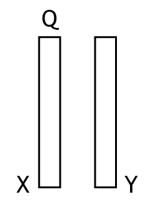Solution:

(a) Charge present on the plate is Q …(Given)

So due to convection, other plate will get the same charge i.e. Q.

Let σ1 and σ2 the surface charge densities on each side of the plate.

Electric field due to a plate = E = σ/2Єo

So, magnitudes of the electric fields become: σ1/2Єo and σ2/2Єo

The charge on each side = q1 = q2 = Q/2

=> Net surface charge density on each side = Q/2A

Where Area of plate = A.

(b) Electric field to the left of the plates

Charge density on the left side of the plate surface = σ = Q/2A

So, electric field = Q/2AЄo

As “x” is positively-charged plate, so above result must be directed towards the left

(c) Charged plate “x” acts as the only source of electric field, with positive in the inner side. A negative charge will be induced on inner side of plate Y, as it is neutral and plate Y attracts the charged particle towards itself. The middle portion E is towards the right and is equal to Q/2AЄo

d) The outer side of plate ‘Y’ acts as positive and hence it repels to the right with E = Q/2AЄo

Question 24: Three identical metal plates with large surface areas are kept parallel to each other as shown in figure. The leftmost plate is given a charge Q, the rightmost a charge –2Q and the middle one remains neutral. Find the charge appearing on the outer surface of the rightmost plate.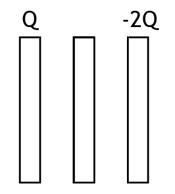Solution:

Let “Q” be the charge on the left plate and “-2Q” be the charge on the rightmost plate.

Two faces of this closed surface lie completely in the conductor, where the electric field is zero. The other parts of the closed surface that are outside are parallel to the electric field and therefore the flow on these parts is zero. The total flow of the electric field through this closed surface is zero. According to Gauss’s law, the total charge within the closed surface is zero.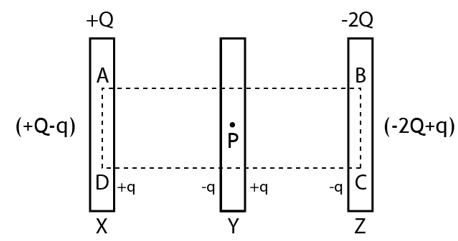The electric field due to a thin plate of charge Q : E = Q/2AЄo

Electric field at point P:

Due to outer surface of plate “X” towards right= (Q-q)/2AЄo

Due to inner surface of plate “X” towards right= q/2AЄo

Due to right surface of plate “Y” towards left = q/2AЄo

Due to Left surface of plate “Y” toward left towards left = q/2AЄo

Due to outer surface of plate “Z” towards right = (2Q-q)/2AЄo

Due to inner surface of plate “Z” towards right = q/2AЄo

Now, Net field at point P: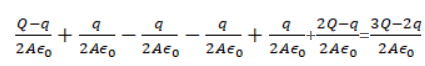[By considering field towards right as positive and towards left as negative]

We know, field inside a point inside conductor = 0.

(3Q-2q)/2AЄo = 0

=> q = 3Q/2

The final charge distribution as: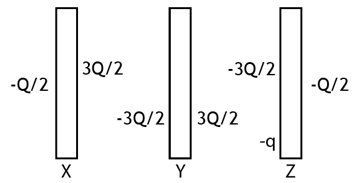Charge on the outer surface of rightmost plate = -2Q + q = -2Q +3Q/2 = -Q/2